# Kumari-Dass equation of state

Kumari and Dass presented a model based on a linear bulk modulus equation, in the spirit of the Murnaghan equation of state. The equation of state does not correctly model the bulk modulus as the pressure,$p$, tends towards infinity, as it remains bounded. This is apparent in the equation relating the bulk modulus to pressure: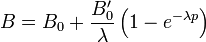$B=B_0+\frac{B_0'}{\lambda}\left(1-e^{-\lambda p}\right)$
where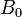$B_0$ is the isothermal bulk modulus,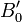$B_0'$ is the pressure derivative of the bulk modulus and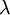$\lambda$ is a softening parameter for the bulk modulus. This leads to a equation for pressure dependent on these parameters of the form: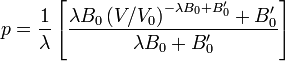$p=\frac{1}{\lambda}\left[\frac{\lambda B_0 \left(V/V_0\right)^{-\lambda B_0 + B_0'}+B_0'}{\lambda B_0 + B_0'}\right]$